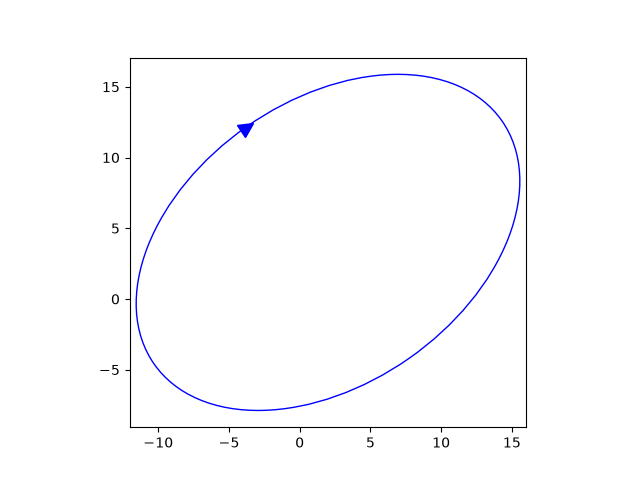# Ellipse with orientation arrow demo#

This demo shows how to draw an ellipse with an orientation arrow (clockwise or counterclockwise). Compare this to the Ellipse collection example.

import matplotlib.pyplot as plt

from matplotlib.markers import MarkerStyle
from matplotlib.patches import Ellipse
from matplotlib.transforms import Affine2D

# Create a figure and axis
fig, ax = plt.subplots(subplot_kw={"aspect": "equal"})

ellipse = Ellipse(
xy=(2, 4),
width=30,
height=20,
angle=35,
facecolor="none",
edgecolor="b"
)

# Plot an arrow marker at the end point of minor axis
vertices = ellipse.get_co_vertices()
t = Affine2D().rotate_deg(ellipse.angle)
ax.plot(
vertices,
vertices,
color="b",
marker=MarkerStyle(">", "full", t),
markersize=10
)
# Note: To reverse the orientation arrow, switch the marker type from > to <.

plt.show()References

The use of the following functions, methods, classes and modules is shown in this example:

Gallery generated by Sphinx-Gallery